# Random Forest and XGBoost hyperparameter tuning and interpretation¶

Follow along for examples of training and tuning random forest and gradient boosting algorithms on popular ML datasets.

In Section 1, we'll compare a set of default parameters for XGBoost, random forest, CatBoost and Gaussian naive bayes over a set of ML competition datasets.

Section 2 will demonstrate tuning the hyperparameters of the random forest, followed by random forest interpretation.

Section 3 extends Section 2 to XGBoost. We'll reproduce aspects of feature selection and hyperparameter tuning from Section 2 with XGBoost. Interpretation follows.

This demonstration largely follows fastai's machine learning tutorial and Analytics Vidhya's guide to XGBoost tuning.

In :
%load_ext autoreload

In :
# helper function library
%run -i '~/pylib.py'

In :
import pandas as pd
import numpy as np
import xgboost as xgb
from xgboost.sklearn import XGBClassifier, XGBRegressor
from catboost import CatBoostClassifier, Pool

from sklearn.model_selection import train_test_split, GridSearchCV
from sklearn import cross_validation, metrics #Additional scklearn functions
from sklearn.datasets import fetch_california_housing
from sklearn.linear_model import LogisticRegression
from sklearn.naive_bayes import GaussianNB
from sklearn.ensemble import RandomForestClassifier, RandomForestRegressor, forest
from sklearn.tree import export_graphviz
from sklearn.model_selection import ParameterGrid
from sklearn.metrics import roc_auc_score

from IPython.display import display
import graphviz
import seaborn as sb

import matplotlib.pylab as plt
%matplotlib inline
from matplotlib.pylab import rcParams
rcParams['figure.figsize'] = 12, 4

from pmlb import fetch_data, classification_dataset_names

import pprint

# import warnings filter
from warnings import simplefilter
# ignore all future warnings
simplefilter(action='ignore', category=FutureWarning)

/Library/Frameworks/Python.framework/Versions/3.6/lib/python3.6/site-packages/IPython/core/interactiveshell.py:3049: DtypeWarning: Columns (13,39,40,41) have mixed types. Specify dtype option on import or set low_memory=False.
interactivity=interactivity, compiler=compiler, result=result)


## Gathering datasets¶

We'll start by gathering datasets that we can perform binary and multiple classification on. We'll use datasets from the Penn Machine Learning Benchmark, a collection of real-world benchmark datasets commonly used in ML benchmarking studies.

In :
from pmlb import classification_dataset_names

# All the classifications in the archive
print(classification_dataset_names)
# The subset of datasets we'll use
classifications_to_use = ['adult', 'heart-c', 'heart-h', 'breast-w', 'iris', 'australian', 'glass']

['GAMETES_Epistasis_2-Way_1000atts_0.4H_EDM-1_EDM-1_1', 'GAMETES_Epistasis_2-Way_20atts_0.1H_EDM-1_1', 'GAMETES_Epistasis_2-Way_20atts_0.4H_EDM-1_1', 'GAMETES_Epistasis_3-Way_20atts_0.2H_EDM-1_1', 'GAMETES_Heterogeneity_20atts_1600_Het_0.4_0.2_50_EDM-2_001', 'GAMETES_Heterogeneity_20atts_1600_Het_0.4_0.2_75_EDM-2_001', 'Hill_Valley_with_noise', 'Hill_Valley_without_noise', 'adult', 'agaricus-lepiota', 'allbp', 'allhyper', 'allhypo', 'allrep', 'analcatdata_aids', 'analcatdata_asbestos', 'analcatdata_authorship', 'analcatdata_bankruptcy', 'analcatdata_boxing1', 'analcatdata_boxing2', 'analcatdata_creditscore', 'analcatdata_cyyoung8092', 'analcatdata_cyyoung9302', 'analcatdata_dmft', 'analcatdata_fraud', 'analcatdata_germangss', 'analcatdata_happiness', 'analcatdata_japansolvent', 'analcatdata_lawsuit', 'ann-thyroid', 'appendicitis', 'australian', 'auto', 'backache', 'balance-scale', 'banana', 'biomed', 'breast', 'breast-cancer', 'breast-cancer-wisconsin', 'breast-w', 'buggyCrx', 'bupa', 'calendarDOW', 'car', 'car-evaluation', 'cars', 'cars1', 'chess', 'churn', 'clean1', 'clean2', 'cleve', 'cleveland', 'cleveland-nominal', 'cloud', 'cmc', 'coil2000', 'colic', 'collins', 'confidence', 'connect-4', 'contraceptive', 'corral', 'credit-a', 'credit-g', 'crx', 'dermatology', 'diabetes', 'dis', 'dna', 'ecoli', 'fars', 'flags', 'flare', 'german', 'glass', 'glass2', 'haberman', 'hayes-roth', 'heart-c', 'heart-h', 'heart-statlog', 'hepatitis', 'horse-colic', 'house-votes-84', 'hungarian', 'hypothyroid', 'ionosphere', 'iris', 'irish', 'kddcup', 'kr-vs-kp', 'krkopt', 'labor', 'led24', 'led7', 'letter', 'liver-disorder', 'lupus', 'lymphography', 'magic', 'mfeat-factors', 'mfeat-fourier', 'mfeat-karhunen', 'mfeat-morphological', 'mfeat-pixel', 'mfeat-zernike', 'mnist', 'mofn-3-7-10', 'molecular-biology_promoters', 'monk1', 'monk2', 'monk3', 'movement_libras', 'mushroom', 'mux6', 'new-thyroid', 'nursery', 'optdigits', 'page-blocks', 'parity5', 'parity5+5', 'pendigits', 'phoneme', 'pima', 'poker', 'postoperative-patient-data', 'prnn_crabs', 'prnn_fglass', 'prnn_synth', 'profb', 'promoters', 'ring', 'saheart', 'satimage', 'schizo', 'segmentation', 'shuttle', 'sleep', 'solar-flare_1', 'solar-flare_2', 'sonar', 'soybean', 'spambase', 'spect', 'spectf', 'splice', 'tae', 'texture', 'threeOf9', 'tic-tac-toe', 'titanic', 'tokyo1', 'twonorm', 'vehicle', 'vote', 'vowel', 'waveform-21', 'waveform-40', 'wdbc', 'wine-quality-red', 'wine-quality-white', 'wine-recognition', 'xd6', 'yeast']


We'll also use Kaggle's "Blue Book for Bulldozers", a popular competition dataset, in Sections 2 and 3.

In [ ]:
PATH = './data/bulldozers/'


## Section 1: Fitting multiple models with defaults¶

First, we'll define some functions to setup our default models with XGBoost and Catboost.

Our Random Forest classifier will be assigned in the model fit loop.

In [ ]:
# Our default Catboost model
def Catmodelfit(X, y):
X_train,X_test,y_train,y_test= train_test_split(X, y, test_size=0.2, random_state=123)

params = {
'iterations':2,
'depth':2,
'learning_rate':1,
'loss_function':'Logloss',
'verbose':True
}

if (len(np.unique(y)) > 2):
params['loss_function'] = 'MultiClass'

model = CatBoostClassifier()
model.set_params(**params)
# train the model
model.fit(X_train, y_train)
return model.score(X_test,y_test)

In [ ]:
# Our default XGBoost model
def modelfit(alg, X, y, useTrainCV=True, cv_folds=5, early_stopping_rounds=50):

X_train,X_test,y_train,y_test= train_test_split(X, y, test_size=0.2, random_state=123)

if useTrainCV:
xgb_param = alg.get_xgb_params()
xgtrain = xgb.DMatrix(X_train, label=y_train)
cvresult = xgb.cv(xgb_param, xgtrain, num_boost_round=alg.get_params()['n_estimators'],
nfold=cv_folds, early_stopping_rounds=early_stopping_rounds)
alg.set_params(n_estimators=cvresult.shape)

#Fit the algorithm on the data
alg.fit(X_train, y_train, eval_metric='auc')

#Predict training set:
dtrain_predictions = alg.predict(X_test)
dtrain_predprob = alg.predict_proba(X_test)[:,1]

#Print model report:
print("\nModel Report")
print("Accuracy : %.4g" % metrics.accuracy_score(y_test, dtrain_predictions))
#print("AUC Score (Train): %f" % metrics.roc_auc_score(y_test, dtrain_predprob))

feat_imp = pd.Series(alg.get_booster().get_fscore()).sort_values(ascending=False)
feat_imp.plot(kind='bar', title='Feature Importances')
plt.ylabel('Feature Importance Score')
return metrics.accuracy_score(y_test, dtrain_predictions)


This is our training loop. We iterate through the subset of ML datasets, appending the scores of the trained models on our test sets to a list.

In [ ]:
logit_test_scores = []
gnb_test_scores = []
gbm_test_scores = []
rf_test_scores = []
cb_test_scores = []

for classification_dataset in classifications_to_use:
X, y = fetch_data(classification_dataset, return_X_y=True)
train_X, test_X, train_y, test_y = train_test_split(X, y)
'''
SKLearn defaults
'''
# logit = LogisticRegression()
gnb = GaussianNB()
rf = RandomForestClassifier()

params = {
'learning_rate':0.05,
'n_estimators':1000,
'max_depth':9,
'min_child_weight':5,
'gamma':0,
'subsample':0.8,
'colsample_bytree':0.8,
'objective': 'binary:logistic',
'scale_pos_weight':1,
'seed':27
}

if (len(np.unique(y)) > 2):
params['num_class'] = np.amax(y).item() + 1
params['objective'] = 'multi:softprob'

xgb1 = XGBClassifier()
xgb1.set_params(**params)

#logit.fit(train_X, train_y)
gnb.fit(train_X, train_y)
rf.fit(train_X, train_y)
gbm_test_scores.append(modelfit(xgb1, X, y))
cb_test_scores.append(Catmodelfit(X, y))

#logit_test_scores.append(logit.score(test_X, test_y))
gnb_test_scores.append(gnb.score(test_X, test_y))
rf_test_scores.append(rf.score(test_X, test_y))


Our results are shown in boxplots.

Random forest has the most consistent estimates. XGBoost's median is the highest (but has the widest confidence interval spread). We'll show in Sections 2 and 3 how we can tune the Random Forest and XGBoost to increase prediction accuracy.

In :
sb.boxplot(data=[gnb_test_scores, gbm_test_scores, rf_test_scores, cb_test_scores], notch=True)
plt.xticks([0, 1, 2, 3], ['GaussianNB', 'GB', 'RF', 'Catboost'])
plt.ylabel('Test Accuracy')

Out:
Text(0, 0.5, 'Test Accuracy')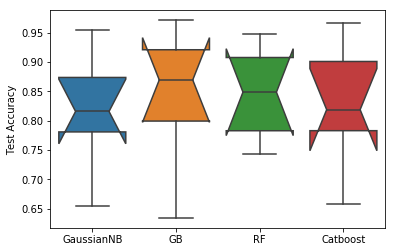## Section 2: RF tuning and interpretation¶

Let's explore how a Random Forest estimate can be improved. Let's also interpret the results more closely.

In :
# We'll use some helpers from fastai
# Other helpers not in fastai v1 are in '~/pylib.py'
from fastai.imports  import *
from fastai import *


Let's take a look a the dataset we'll be using: the bulldozers dataset imported above.

In :
display_all(df_raw.tail().T)

401120 401121 401122 401123 401124
SalesID 6333336 6333337 6333338 6333341 6333342
SalePrice 10500 11000 11500 9000 7750
MachineID 1840702 1830472 1887659 1903570 1926965
ModelID 21439 21439 21439 21435 21435
datasource 149 149 149 149 149
auctioneerID 1 1 1 2 2
YearMade 2005 2005 2005 2005 2005
MachineHoursCurrentMeter NaN NaN NaN NaN NaN
UsageBand NaN NaN NaN NaN NaN
saledate 2011-11-02 00:00:00 2011-11-02 00:00:00 2011-11-02 00:00:00 2011-10-25 00:00:00 2011-10-25 00:00:00
fiModelDesc 35NX2 35NX2 35NX2 30NX 30NX
fiBaseModel 35 35 35 30 30
fiSecondaryDesc NX NX NX NX NX
fiModelSeries 2 2 2 NaN NaN
fiModelDescriptor NaN NaN NaN NaN NaN
ProductSize Mini Mini Mini Mini Mini
fiProductClassDesc Hydraulic Excavator, Track - 3.0 to 4.0 Metric... Hydraulic Excavator, Track - 3.0 to 4.0 Metric... Hydraulic Excavator, Track - 3.0 to 4.0 Metric... Hydraulic Excavator, Track - 2.0 to 3.0 Metric... Hydraulic Excavator, Track - 2.0 to 3.0 Metric...
state Maryland Maryland Maryland Florida Florida
ProductGroup TEX TEX TEX TEX TEX
ProductGroupDesc Track Excavators Track Excavators Track Excavators Track Excavators Track Excavators
Drive_System NaN NaN NaN NaN NaN
Enclosure EROPS EROPS EROPS EROPS EROPS
Forks NaN NaN NaN NaN NaN
Pad_Type NaN NaN NaN NaN NaN
Ride_Control NaN NaN NaN NaN NaN
Stick NaN NaN NaN NaN NaN
Transmission NaN NaN NaN NaN NaN
Turbocharged NaN NaN NaN NaN NaN
Blade_Extension NaN NaN NaN NaN NaN
Blade_Width NaN NaN NaN NaN NaN
Enclosure_Type NaN NaN NaN NaN NaN
Engine_Horsepower NaN NaN NaN NaN NaN
Hydraulics Auxiliary Standard Auxiliary Standard Standard
Pushblock NaN NaN NaN NaN NaN
Ripper NaN NaN NaN NaN NaN
Scarifier NaN NaN NaN NaN NaN
Tip_Control NaN NaN NaN NaN NaN
Tire_Size NaN NaN NaN NaN NaN
Coupler None or Unspecified None or Unspecified None or Unspecified None or Unspecified None or Unspecified
Coupler_System NaN NaN NaN NaN NaN
Grouser_Tracks NaN NaN NaN NaN NaN
Hydraulics_Flow NaN NaN NaN NaN NaN
Track_Type Steel Steel Steel Steel Steel
Undercarriage_Pad_Width None or Unspecified None or Unspecified None or Unspecified None or Unspecified None or Unspecified
Stick_Length None or Unspecified None or Unspecified None or Unspecified None or Unspecified None or Unspecified
Thumb None or Unspecified None or Unspecified None or Unspecified None or Unspecified None or Unspecified
Pattern_Changer None or Unspecified None or Unspecified None or Unspecified None or Unspecified None or Unspecified
Grouser_Type Double Double Double Double Double
Backhoe_Mounting NaN NaN NaN NaN NaN
Blade_Type NaN NaN NaN NaN NaN
Travel_Controls NaN NaN NaN NaN NaN
Differential_Type NaN NaN NaN NaN NaN
Steering_Controls NaN NaN NaN NaN NaN

Let's add some useful features to the dataset. This is a time-series dataset, so we want to be careful how we validate and stratify the datset by date features.

In :
# Add "date parts": Columns with features from the time-series
# For example, we've added a saleYear column

Out:
0    2006
1    2004
2    2004
3    2011
4    2009
Name: saleYear, dtype: int64

Now let's convert string variables in the dataset to numeric values. train_cats traverses the dataset, finding columns with strings and transforming them to factors.

In :
train_cats(df_raw)


We can also manually define factors for interpretability.

In :
df_raw.UsageBand.cat.set_categories(['High', 'Medium', 'Low'], ordered=True, inplace=True)


Save the pre-processed dataset for future use.

In :
os.makedirs('tmp', exist_ok=True)
df_raw.to_feather('tmp/bulldozers-raw')


In :
df_raw = pd.read_feather('tmp/bulldozers-raw')


Fastai has a nice function to replace categories with their numeric codes, handle missing continuous values, and split the dependent variable into a separate variable. We've already done a lot of that pre-processing, but let's perform it here anyway to deal with NA values and create a separate dataframe for our predictor and response columns.

In :
df, y, nas = proc_df(df_raw, 'SalePrice')


### Initial training and tuning (overfit)¶

To start, we'll train a preliminary model. We obtain a prediction score of 0.856, using the default scikit-learn RF hyperparameters. We use OOB scoring and do not predict on the test set yet. We'll later define a print score function to print multiple error evaluations.

In :
def split_vals(a,n): return a[:n].copy(), a[n:].copy()

n_valid = 12000  # same as Kaggle's test set size
n_trn = len(df)-n_valid

X_train, X_valid = split_vals(df, n_trn)
y_train, y_valid = split_vals(y, n_trn)
raw_train, raw_valid = split_vals(df_raw, n_trn)
df_trn, y_trn, nas = proc_df(df_raw, 'SalePrice')


Our full model and a model trained on a subset of data for each tree provides a baseline to compre against.

In :
m = RandomForestRegressor(n_jobs=-1, oob_score=True)

%time m.fit(X_train, y_train)
print(m.oob_score_)

CPU times: user 1min 43s, sys: 4.21 s, total: 1min 47s
Wall time: 53.6 s
0.8514041289927369

In :
set_rf_samples(20000)

m = RandomForestRegressor(n_jobs=-1, oob_score=True)

%time m.fit(X_train, y_train)
print(m.oob_score_)

CPU times: user 10.6 s, sys: 929 ms, total: 11.5 s
Wall time: 7.77 s
0.8558677230783271


Our first hyperparameter to tune is n_estimators: the number of trees in the forest. We can increase the number to improve our model performance.

In :
m = RandomForestRegressor(n_estimators=40, n_jobs=-1, oob_score=True)

%time m.fit(X_train, y_train)
print(m.oob_score_)

CPU times: user 29.5 s, sys: 2.04 s, total: 31.5 s
Wall time: 18.5 s
0.8716851397422587


Increasing again, we get a slight improvement. We'll tune max_features and min_samples_leaf parameters to reduce overfitting. max_features restricts the features we sample from in each tree to a subset of all possible features. min_samples_leaf sets the minimum number of samples necessary to continue branching the tree before reaching a leaf node.

In :
m = RandomForestRegressor(n_estimators=120, n_jobs=-1, oob_score=True)

%time m.fit(X_train, y_train)
print(m.oob_score_)

CPU times: user 1min 24s, sys: 4.96 s, total: 1min 29s
Wall time: 51.2 s
0.8747933802034709

In :
m = RandomForestRegressor(n_estimators=40, min_samples_leaf=3, max_features=0.5,
n_jobs=-1, oob_score=True)
%time m.fit(X_train, y_train)
print(m.oob_score_)

CPU times: user 33.9 s, sys: 3.28 s, total: 37.2 s
Wall time: 26.2 s
0.8656095413415806


Grid search is an automated method for searching for hyperparameters that improve our model performance. It tries multiple models simultaneously. The idea is that there should be an optimal hyperparamter set that can be discovered by trying all possible combinations. Regularization can be added to prevent overfitting.

Grid search is something I plan to return to in the context of RF. I found I often run out of memory performing it on the full dataset. When performing on a smaller subset, the results do not always translate to the full dataset. Here, we find that 0.3 is our optimal parameter for max_features. Jeremy uses 0.5, which we'll stick with for the rest of the examples.

In :
df_trn_, y_trn_, nas = proc_df(df_raw, 'SalePrice', subset=30000, max_n_cat=7)
X_train, _ = split_vals(df_trn_, 20000)
y_train, _ = split_vals(y_trn_, 20000)

paramGrid = {
'min_samples_leaf': ,
'max_features': ['log2', 'sqrt', 0.3, 0.5, 0.7],
'n_estimators': 
}

rfc=RandomForestRegressor(random_state=42, n_jobs=-1, oob_score = True)

CV_rfc = GridSearchCV(estimator=rfc, param_grid=paramGrid, cv= 5)
CV_rfc.fit(X_train, y_train)

print(CV_rfc.best_params_)

{'max_features': 0.3, 'min_samples_leaf': 3, 'n_estimators': 100}


#### Confidence interval estimation¶

The basic technique for CI estimation is

In :
def parallel_trees(m, fn, n_jobs=8):
return list(ProcessPoolExecutor(n_jobs).map(fn, m.estimators_))

In :
def get_preds(t): return t.predict(X_valid)
%time preds = np.stack(parallel_trees(m, get_preds))
np.mean(preds[:,0]), np.std(preds[:,0])

CPU times: user 194 ms, sys: 201 ms, total: 395 ms
Wall time: 3.05 s

Out:
(11487.083333333334, 5141.435013014903)
In :
pd.options.display.max_columns = None
print(y[:500])

[66000 57000 10000 38500 ... 17000 33000 16500 70000]


We can examine a confidence interval for our estimates of different enclosure types' sale price. We see some enclosers are easier to estimate.

In :
x = raw_valid.copy()
x['pred_std'] = np.std(preds, axis=0)
x['pred'] = np.mean(preds, axis=0)
flds = ['Enclosure', 'SalePrice', 'pred', 'pred_std']
enc_summ = x[flds].groupby('Enclosure', as_index=False).mean()
enc_summ = enc_summ[~pd.isnull(enc_summ.SalePrice)]
enc_summ.plot('Enclosure', 'pred', 'barh', xerr='pred_std', alpha=0.6, xlim=(0,75000));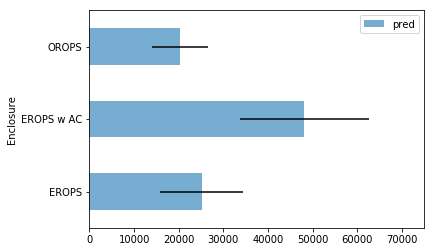The addition of min_samples_leaf and max_features helps us reduce overfitting without much cost to our model accuracy. As we learn more about our data, we can take additional steps to reduce overfitting to the data.

First, we can remove features that are not contributing very much to the model and are likely just noisy indicators. This also allows us to interpret which features are most important to predicting SalePrice, our response column.

#### Remove unnecessary features¶

In [ ]:
def rf_feat_importance(m, df):
return pd.DataFrame({'cols':df.columns, 'imp':m.feature_importances_}
).sort_values('imp', ascending=False)

In :
m = RandomForestRegressor(n_estimators=60, min_samples_leaf=3, max_features=0.5,
n_jobs=-1, oob_score=True)
%time m.fit(X_train, y_train)

CPU times: user 31.9 s, sys: 2.53 s, total: 34.4 s
Wall time: 22.6 s

Out:
RandomForestRegressor(bootstrap=True, criterion='mse', max_depth=None,
max_features=0.5, max_leaf_nodes=None,
min_impurity_decrease=0.0, min_impurity_split=None,
min_samples_leaf=3, min_samples_split=2,
min_weight_fraction_leaf=0.0, n_estimators=60, n_jobs=-1,
oob_score=True, random_state=None, verbose=0, warm_start=False)
In :
fi = rf_feat_importance(m, df_trn); fi[:10]

Out:
cols imp
13 ProductSize 0.141624
19 Enclosure 0.071801
10 fiSecondaryDesc 0.068337
63 saleElapsed 0.057751
14 fiProductClassDesc 0.050600
2 ModelID 0.046803
8 fiModelDesc 0.040721
12 fiModelDescriptor 0.032580
9 fiBaseModel 0.028785

Clearly, the year made, enclosure type and size of the bulldozer tell us a lot about how much the bulldozer sells for. That agrees with our expectations.

In :
fi.plot('cols', 'imp', figsize=(10,6), legend=False);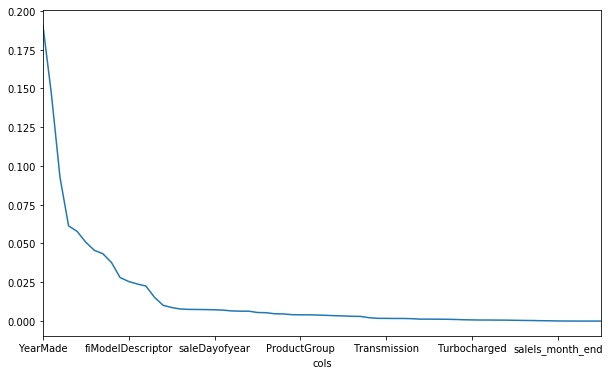We keep a few dozen features from the original set.

In :
to_keep = fi[fi.imp>0.005].cols; len(to_keep)

Out:
28
In :
df_keep = df[to_keep].copy()
X_train, X_valid = split_vals(df_keep, n_trn)
y_train, y_valid = split_vals(y, n_trn)


Even reducing our feature set, with our tuned hyperparameters, our oob score exceeds that of our original model with the full feature set.

In :
set_rf_samples(20000)
m = RandomForestRegressor(n_estimators=60, min_samples_leaf=3, max_features=0.5,
n_jobs=-1, oob_score=True)
m.fit(X_train, y_train)
print(m.oob_score_)

0.8686036464989187


#### Remove correlated features¶

Next we'll remove collinear features (features that provide roughly the same information to the model). This also yields some insight into where interactions between data points are occurring.

In :
from scipy.cluster import hierarchy as hc

corr = np.round(scipy.stats.spearmanr(df_keep).correlation, 4)
corr_condensed = hc.distance.squareform(1-corr)
fig = plt.figure(figsize=(16,10))
dendrogram = hc.dendrogram(z, labels=df_keep.columns, orientation='left', leaf_font_size=16)
plt.show()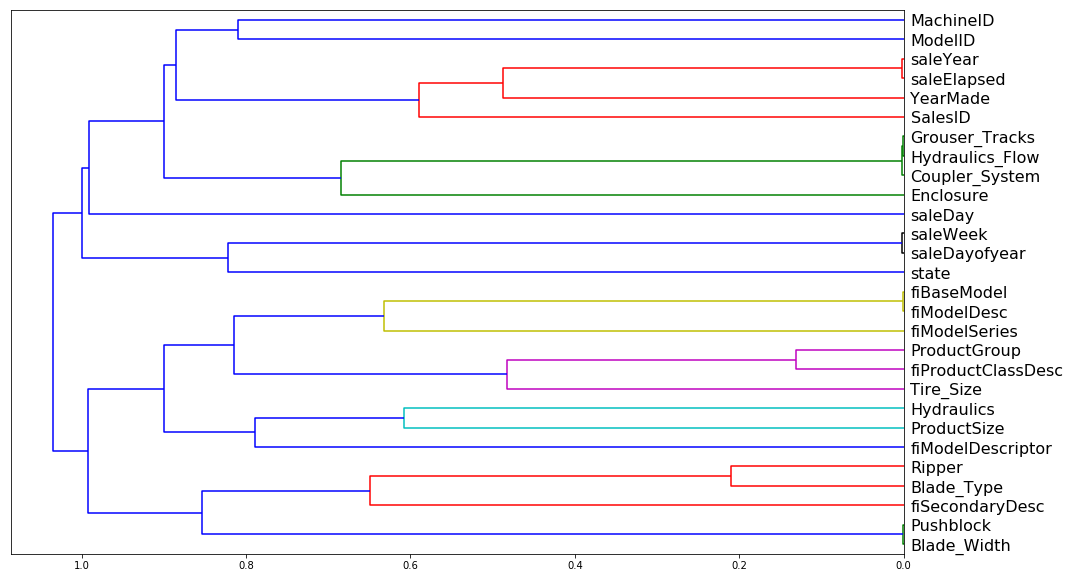In :
def get_oob(df):
m = RandomForestRegressor(n_estimators=60, min_samples_leaf=3, max_features=0.5, n_jobs=-1, oob_score=True)
x, _ = split_vals(df, n_trn)
m.fit(x, y_train)
return m.oob_score_


We can look through a few rank correlated features. I got different values here than Jeremy does in his fastai tutorial. That's likely do to the variation in random sampling.

In :
for c in ('saleYear', 'saleElapsed', 'SalesID', 'YearMade'):
print(c, get_oob(df_keep.drop(c, axis=1)))

saleYear 0.865798626162252
saleElapsed 0.8623029217403086
SalesID 0.8674013209917741


We'll drop two columns that don't appera to impact our scoring.

In :
to_drop = ['saleYear', 'SalesID']
get_oob(df_keep.drop(to_drop, axis=1))

In :
df_keep.drop(to_drop, axis=1, inplace=True)
X_train, X_valid = split_vals(df_keep, n_trn)


Save our column set. Now, without collinear columns, low importance columns and with transformations and NA values addressed.

In :
np.save('tmp/keep_cols.npy', np.array(df_keep.columns))

In :
keep_cols = np.load('tmp/keep_cols.npy', allow_pickle=True)
df_keep = df_trn[keep_cols]

In :
X_train, X_valid = split_vals(df_keep, n_trn)
y_train, y_valid = split_vals(y, n_trn)


Reset our RF sample size to the full dataset. Our model performs well (last column is oob score; the second to last is our test set).

In :
reset_rf_samples()


We create a function to print the score on our test dataset and oob score.

In [ ]:
def rmse(x,y): return math.sqrt(((x-y)**2).mean())

# score returns the r square
def print_score(m):
res = [rmse(m.predict(X_train), y_train), rmse(m.predict(X_valid), y_valid),
m.score(X_train, y_train), m.score(X_valid, y_valid)]
if hasattr(m, 'oob_score_'): res.append(m.oob_score_)
print(res)


Our final model performs well. The oob score (last array entry) and test score (second to last) is above that of our initial model, while we have taken steps to reduce overfitting.

We can grid search over the size of the forest with the full dataset to try to improve the score further.

In :
paramGrid = {
'n_estimators': [100, 500, 1000]
}

rfc=RandomForestRegressor(min_samples_leaf=3, max_features=0.5, random_state=42, n_jobs=-1, oob_score = True)

CV_rfc = GridSearchCV(estimator=rfc, param_grid=paramGrid, cv=5)
CV_rfc.fit(X_train, y_train)

print_score(CV_rfc)

[4130.916847815229, 7504.800307967265, 0.9677355464683458, 0.9042155047300625]

In :
print(CV_rfc.best_params_)

{'n_estimators': 1000}


A large forest does perform better, but not by much. Our 160 tree forest actually still beats our 5,000 tree forest on the test dataset. The larger forest does win on the OOB error.

In :
m = RandomForestRegressor(n_estimators=160, min_samples_leaf=3, max_features=0.5, n_jobs=-1, oob_score=True)
m.fit(X_train, y_train)
print_score(m)

[4228.339551131074, 7435.621054121741, 0.966195764497023, 0.9059732488468275, 0.9125273056583695]

In :
m = RandomForestRegressor(n_estimators=1000, min_samples_leaf=3, max_features=0.5, n_jobs=-1, oob_score=True)
m.fit(X_train, y_train)
print_score(m)

[4215.725227997296, 7451.82617237472, 0.9663971586770245, 0.9055629603196176, 0.9135018023890233]

In :
m = RandomForestRegressor(n_estimators=5000, min_samples_leaf=3, max_features=0.5, n_jobs=-1, oob_score=True)
m.fit(X_train, y_train)
print_score(m)

[4210.9860632315995, 7445.381457584097, 0.9664726663924605, 0.9057262375055872, 0.9137638844855438]


#### Partial dependence¶

Next, let's use partial dependence analysis to look at the impact of some of the most important features more closely. Partial dependence is similar to multiple regression. It determines the effect of individual predictors on our response, holding all other predictors constant.

In :
from pdpbox import pdp
from plotnine import *

In :
set_rf_samples(50000)

In :
df_trn2, y_trn, nas = proc_df(df_raw, 'SalePrice', max_n_cat=7)
X_train, X_valid = split_vals(df_trn2, n_trn)
m = RandomForestRegressor(n_estimators=40, min_samples_leaf=3, max_features=0.5, n_jobs=-1)
m.fit(X_train, y_train);

In :
x = get_sample(X_train[X_train.YearMade>1930], 500)

In :
def plot_pdp(feat_name, clusters=None):
feat_name = feat_name or feat
p = pdp.pdp_isolate(m, x, feature=feat_name, model_features=x.columns)
return pdp.pdp_plot(p, feat_name, plot_lines=True,
cluster=clusters is not None, n_cluster_centers=clusters)


What we see is that there's a close-to-linear relationship between year made and sale price. If we did not adjust for sampling in our data, we might have seen a rising and falling line over the late 1980s. But this would have been the result of other variables influencing the Saleprice during that period. Controling for those effects, we see a fairly linear relationship.

In :
plot_pdp('YearMade')

Out:
(<Figure size 1080x684 with 2 Axes>,
'pdp_ax': <matplotlib.axes._subplots.AxesSubplot at 0x14ac52a20>})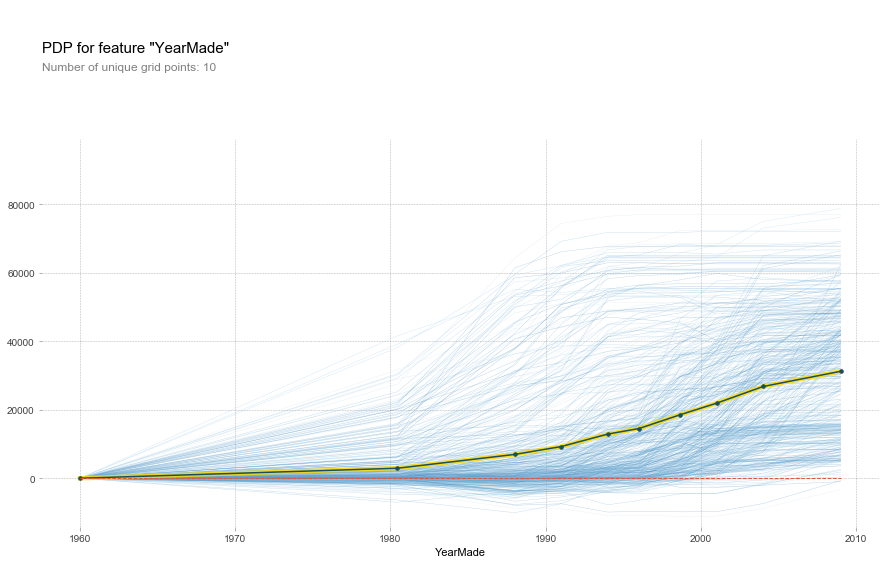features_to_plot = ['saleElapsed', 'YearMade']